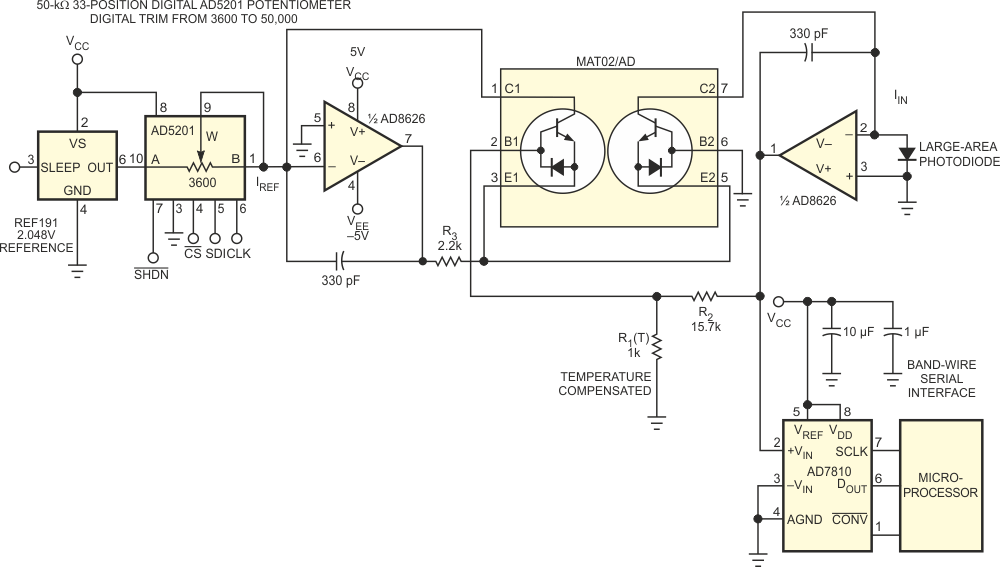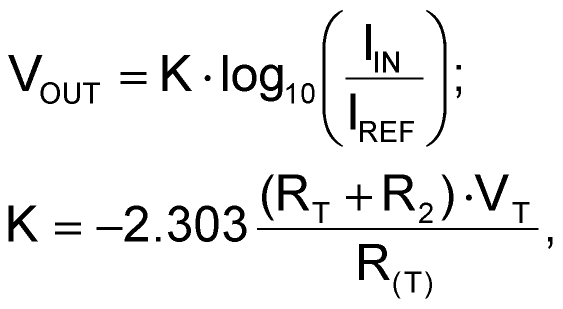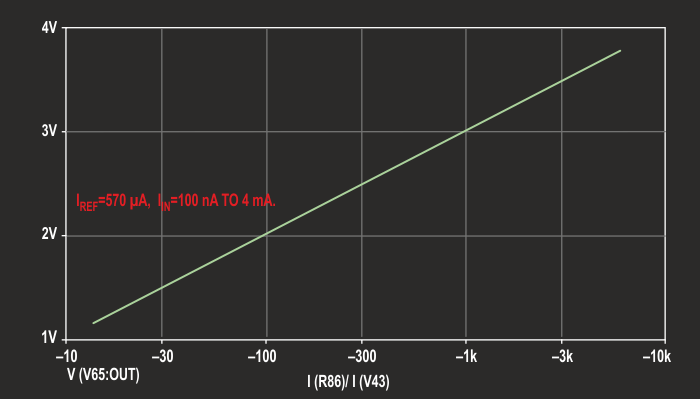# Log-ratio amplifier has six-decade dynamic range

Reza Moghimi

EDN

You need optical-power monitoring to guarantee overall system performance in fiber-optic communication systems. Logarithmic-signal processing can maintain precise measurements over a wide dynamic range. The wide-dynamic-range signal undergoes compression, and the use of a lower resolution measurement system then saves cost. As an example of this technique, consider a photodiode with responsivity of 0.5 A/W that converts light energy to a current of 100 nA to 1 mA. With a four-decade dynamic range and 1% error, the required measurement resolution is 0.01×10–4, or 1 ppm. This measurement requires a 20-bit ADC.

Instead, you can compress this input to a 0 to 4 V range using a log-ratio amplifier and then use a 10-bit ADC, substantially reducing the system cost. Programming the reference current allows shifting the output voltage to the desired level. You can customize and use the circuit in Figure 1 in applications involving unusual combinations of dynamic range; input signal, such as voltage or current; polarity; and scaling; or operations such as log products and ratios.Figure 1. This circuit is a programmable, temperature-compensated log-ratio amplifier.

Log-ratio amplifiers find applications in wide-dynamic-range ratiometric measurements, which measure an unknown signal against a variable-current reference. The transfer function of the circuit in Figure 1 is:where K is the output scale factor, IIN is the current that the photodiode generates, VT is a temperature-dependent term (typically, 26 mV at 25 °C and proportional to absolute temperature), and IREF is the reference current. VOUT=0 when IIN=IREF. For proper operation, IIN/IREF should always be greater than 0. The output of the log-ratio circuit can be positive, negative, or bipolar, depending on the ratio of IIN/IREF. The 4 V full-scale input range of the ADC sets the 4-mA full-scale input-current range. Programming IREF to a value of 40 to 600 µA places the output in the middle of the measurement range.

The components give an output-scale factor of –1. This circuit has an output defined over a range of 4.5 decades of signal current, IIN, and 1.5 decades of reference current, IREF (limited by the load-driving capability of the reference for a total six-decade range). For most applications, you would use only a portion of the entire six-decade range. By determining the range of the expected input signals and computing their ratios, you can use the equations to predict the expected output-voltage range. You can assign IREF and IIN to match device performance to the current range, but you should observe polarity.

A log amplifier generally depends on the nonlinear transfer function of a transistor. The general transfer function of a log amplifier is related to IS and VT, which both depend on temperature. IS is the transistor's collector saturation current, and VT is the transistor's "thermal voltage." To overcome this temperature dependency, this design uses a matched pair of MAT02 transistors to cancel the IS temperature drift and a temperature-sensitive resistive voltage divider to compensate for the temperature coefficient of VT. The heart of the IREF generator is a REF191. You adjust its output with an AD5201 digital potentiometer. This modification allows you to program the reference current in 33 steps, from 40 to 600 µA.Figure 2. VOUT has IREF programmed to full scale of 570 µA.Figure 3. VOUT has IREF programmed to zero scale of 40 µA.

The combination of the REF191 and the AD5201 provides a current source that is stable with respect to time and temperature. For higher resolution, you can use the 1024-position AD5231. The AD8626 is a dual precision-JFET-input amplifier with true single-supply operation to 26 V, low power consumption, and rail-to-rail output swing, allowing a wide dynamic range. Its output is stable with capacitive loads in excess of 500 pF. Figure 2 and Figure 3 show the transfer function of the log-ratio amplifier at the input of the ADC. The output is limited to 0 to 4 V to match the unipolar input-voltage range of the AD7810 ADC.

## Reference

1. Sheingold, Dan, Editor, Nonlinear Circuits Handbook, Analog Devices, ISBN: 0-916550-01-X.

## Materials on the topic

EDN

You may have to register before you can post comments and get full access to forum.
 User Name Remember Me? Password